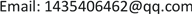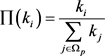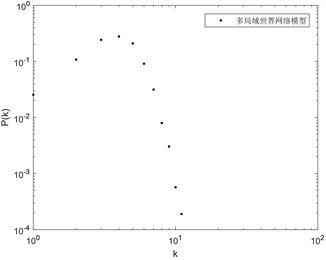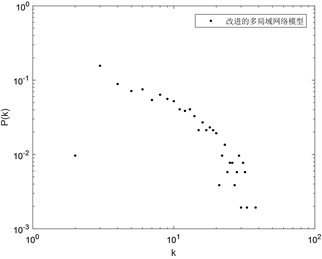﻿ 一种改进的多局域世界网络模型 An Improved Multi-Local-World Network Model

Operations Research and Fuzziology
Vol. 09  No. 04 ( 2019 ), Article ID: 32845 , 7 pages
10.12677/ORF.2019.94033

An Improved Multi-Local-World Network Model

Lulu Zhang

Central University for Nationalities, BeijingReceived: Oct. 16th, 2019; accepted: Oct. 30th, 2019; published: Nov. 6th, 2019ABSTRACT

On the basis of Multi-Local-World network model, this paper introduces the inequality and competitiveness among local networks, constructs an improved Multi-Local-World model, and analyses the degree distribution of the model. The network degree distribution of the model obeys power-law distribution, and the network is scale-free network.

Keywords:Multi-Local-World Network Model, Inequality, Competitiveness1. 引言

2. 模型建立与分析

2.1. 改进的多局域世界模型(IMLW)

1) 以概率 ${p}_{1}$ 增加一个新节点到一个选定的局域网中，且与该局域网中的节点建立 ${n}_{1}$ 条边。首先需要选定一个局域网Ωp，其概率为 $\frac{{r}_{p}}{\underset{q=1}{\overset{m}{\sum }}{r}_{q}}$ (2.11)，接下来新节点与该网络节点i连接的概率为按照度择优的原则(2.12)

2) 以概率 ${p}_{2}$ 增加 ${n}_{2}$ 条边到选定的局域网中。首先需要选首先按照2.11式选定一个局域网 ${\Omega }_{p}$，随机

3) 以概率 ${p}_{3}$ 在选定的局域网中去掉 ${n}_{3}$ 条边。首先需要选定一个局域网 ${\Omega }_{p}$，根据反择优原则，那么一个局域网的竞争力越低被选择的概率就越大。选定局域网Ωp的概率为 $1-\frac{{r}_{p}}{\underset{q=1}{\overset{m}{\sum }}{r}_{q}}$，接下来随机选择一个节点，概率为 $\frac{1}{{N}_{{\Omega }_{p}}\left(t\right)}$，然后反择优选择另一节点，其概率为

${\prod }^{\prime }\left({k}_{i}\right)=\frac{1}{{N}_{{\Omega }_{p}}\left(t\right)-1}\left(1-\prod \left({k}_{i}\right)\right)=\frac{1}{{N}_{{\Omega }_{p}}\left(t\right)-1}\left(1-\frac{{k}_{i}}{\underset{j\in {\Omega }_{p}}{\sum }{k}_{j}}\right)$

4) 以概率 ${p}_{4}$ 选定一个局域网，让其与其它局域网建立 ${n}_{4}$ 条长程边。首先按照2.11选定一个局域网Ωr，然后在该网络中按照2.12式选定一个节点，再按2.11式选择一个局域网Ωp，并在该局域网中按2.12式选择一个节点与之前节点相连，重复上述过程 ${n}_{4}$ 次。

5) 终止条件，运行N步。

2.2. 度分布分析

1) 增加一个带有 ${n}_{1}$ 条边的节点到一个选定的局域网Ωp中。

${\left(\frac{\partial {k}_{i}}{\partial t}\right)}_{1}=\frac{{n}_{1}{p}_{1}{r}_{p}}{\underset{q=1}{\overset{m}{\sum }}{r}_{q}}\frac{{k}_{i}}{\underset{j\in {\Omega }_{p}}{\sum }{k}_{j}}$ (2.13)

2) 增加 ${n}_{2}$ 条边到选定的局域网中。

${\left(\frac{\partial {k}_{i}}{\partial t}\right)}_{2}=\frac{{n}_{2}{p}_{2}{r}_{p}}{\underset{q=1}{\overset{m}{\sum }}{r}_{q}}\left[\frac{1}{{N}_{{\Omega }_{p}}\left(t\right)}+\left(1-\frac{1}{{N}_{{\Omega }_{p}}\left(t\right)}\right)\frac{{k}_{i}}{\underset{j\in {\Omega }_{p}}{\sum }{k}_{j}}\right]$ (2.14)

3) 在选定的局域网中去掉 ${n}_{3}$ 条边。

${\left(\frac{\partial {k}_{i}}{\partial t}\right)}_{3}=-{n}_{3}{p}_{3}\left(1-\frac{{r}_{p}}{\underset{q=1}{\overset{m}{\sum }}{r}_{q}}\right)\left[\frac{1}{{N}_{{\Omega }_{p}}\left(t\right)}+\left(1-\frac{1}{{N}_{{\Omega }_{p}}\left(t\right)}\right)\frac{1}{{N}_{{\Omega }_{p}}\left(t\right)-1}\left(1-\frac{{k}_{i}}{\underset{j\in {\Omega }_{p}}{\sum }{s}_{j}}\right)\right]$ (2.15)

4) 选定一个局域网，让其与其它局域网建立 ${n}_{4}$ 条长程边。

${\left(\frac{\partial {k}_{i}}{\partial t}\right)}_{4}={n}_{4}{p}_{4}\left(\frac{{r}_{p}}{\underset{q=1}{\overset{m}{\sum }}{r}_{q}}\frac{{k}_{i}}{\underset{j\in {\Omega }_{p}}{\sum }{k}_{j}}+\frac{{r}_{j}}{\underset{q=1}{\overset{m}{\sum }}{r}_{q}}\frac{{k}_{i}}{\underset{j\in {\Omega }_{p}}{\sum }{k}_{j}}\right)$ (2.16)

${N}_{{\Omega }_{p}}\left(t\right)={m}_{p}+\frac{{r}_{p}}{\underset{q=1}{\overset{m}{\sum }}{r}_{q}}{p}_{1}t$ (2.17)

$\begin{array}{c}\frac{\partial {k}_{i}}{\partial t}={\left(\frac{\partial {k}_{i}}{\partial t}\right)}_{1}+{\left(\frac{\partial {k}_{i}}{\partial t}\right)}_{2}+{\left(\frac{\partial {k}_{i}}{\partial t}\right)}_{3}+{\left(\frac{\partial {k}_{i}}{\partial t}\right)}_{4}\\ =\frac{{n}_{1}{p}_{1}{r}_{p}}{\underset{q=1}{\overset{m}{\sum }}{r}_{q}}\frac{{k}_{i}}{\underset{j\in {\Omega }_{p}}{\sum }{k}_{j}}+\frac{{n}_{2}{p}_{2}{r}_{p}}{\underset{q=1}{\overset{m}{\sum }}{r}_{q}}\left[\frac{1}{{N}_{{\Omega }_{p}}\left(t\right)}+\left(1-\frac{1}{{N}_{{\Omega }_{p}}\left(t\right)}\right)\frac{{k}_{i}}{\underset{j\in {\Omega }_{p}}{\sum }{k}_{j}}\right]\\ \text{\hspace{0.17em}}\text{\hspace{0.17em}}-{n}_{3}{p}_{3}\left(1-\frac{{r}_{p}}{\underset{q=1}{\overset{m}{\sum }}{r}_{q}}\right)\left[\frac{1}{{N}_{{\Omega }_{p}}\left(t\right)}+\left(1-\frac{1}{{N}_{{\Omega }_{p}}\left(t\right)}\right)\frac{1}{{N}_{{\Omega }_{p}}\left(t\right)-1}\left(1-\frac{{k}_{i}}{\underset{j\in {\Omega }_{p}}{\sum }{k}_{j}}\right)\right]\\ \text{\hspace{0.17em}}\text{\hspace{0.17em}}+{n}_{4}{p}_{4}\left[\frac{{r}_{p}}{\underset{q=1}{\overset{m}{\sum }}{r}_{q}}\frac{{k}_{i}}{\underset{j\in {\Omega }_{p}}{\sum }{k}_{j}}+\frac{{r}_{j}}{\underset{q=1}{\overset{m}{\sum }}{r}_{q}}\frac{{k}_{i}}{\underset{j\in {\Omega }_{p}}{\sum }{k}_{j}}\right]\end{array}$

$\begin{array}{l}=\frac{{n}_{1}{p}_{1}}{c}\cdot \frac{{k}_{i}}{t}+\frac{{n}_{2}{p}_{2}}{{p}_{1}}\cdot \frac{1}{t}+\frac{{n}_{2}{p}_{2}}{c}\cdot \frac{{k}_{i}}{t}-{n}_{2}{p}_{2}\cdot \frac{\underset{q=1}{\overset{m}{\sum }}{r}_{q}}{{r}_{p}c{p}_{1}}\cdot \frac{{k}_{i}}{{t}^{2}}+\frac{{n}_{3}{p}_{3}}{{p}_{1}}\cdot \frac{1}{t}-{n}_{3}{p}_{3}\cdot \frac{\underset{q=1}{\overset{m}{\sum }}{r}_{q}}{{r}_{p}{p}_{1}}\cdot \frac{1}{t}\\ \text{\hspace{0.17em}}\text{\hspace{0.17em}}+{n}_{3}{p}_{3}\cdot \frac{\underset{q=1}{\overset{m}{\sum }}{r}_{q}-{r}_{p}}{\underset{q=1}{\overset{m}{\sum }}{r}_{q}}+{n}_{3}{p}_{3}\cdot \frac{\underset{q=1}{\overset{m}{\sum }}{r}_{q}-{r}_{p}}{c{r}_{p}}\frac{{k}_{i}}{t}+{n}_{3}{p}_{3}\cdot \frac{\left(\underset{q=1}{\overset{m}{\sum }}{r}_{q}-{r}_{p}\right)\cdot \underset{q=1}{\overset{m}{\sum }}{r}_{q}}{{p}_{1}{r}_{p}t\left({p}_{1}{r}_{p}t-\underset{q=1}{\overset{m}{\sum }}{r}_{q}\right)}\\ \text{\hspace{0.17em}}\text{\hspace{0.17em}}+{n}_{3}{p}_{3}\cdot \frac{\left(\underset{q=1}{\overset{m}{\sum }}{r}_{q}-{r}_{p}\right)\cdot {\left(\underset{q=1}{\overset{m}{\sum }}{r}_{q}\right)}^{2}}{c{p}_{1}{r}_{p}t\left({p}_{1}{r}_{p}t-\underset{q=1}{\overset{m}{\sum }}{r}_{q}\right)}\cdot \frac{{k}_{i}}{t}+\frac{2{n}_{4}{p}_{4}}{c}\cdot \frac{{k}_{i}}{t}\\ =\frac{{n}_{1}{p}_{1}}{c}\cdot \frac{{k}_{i}}{t}+\frac{{n}_{2}{p}_{2}}{c}\cdot \frac{{k}_{i}}{t}+{n}_{3}{p}_{3}\cdot \frac{\underset{q=1}{\overset{m}{\sum }}{r}_{q}-{r}_{p}}{c{r}_{p}}\frac{{k}_{i}}{t}+\frac{2{n}_{4}{p}_{4}}{c}\cdot \frac{{k}_{i}}{t}\\ \text{\hspace{0.17em}}\text{\hspace{0.17em}}+\frac{{n}_{2}{p}_{2}}{{p}_{1}}\cdot \frac{1}{t}+\frac{{n}_{3}{p}_{3}}{{p}_{1}}\cdot \frac{1}{t}-{n}_{3}{p}_{3}\cdot \frac{\underset{q=1}{\overset{m}{\sum }}{r}_{q}}{{r}_{p}{p}_{1}}\cdot \frac{1}{t}\end{array}$ (2.20)

$a=\frac{{n}_{1}{p}_{1}}{c}+\frac{{n}_{2}{p}_{2}}{c}+\frac{\underset{q=1}{\overset{m}{\sum }}{r}_{q}-{r}_{p}}{c{r}_{p}}+\frac{2{n}_{4}{p}_{4}}{c}$$b=\frac{{n}_{2}{p}_{2}}{{p}_{1}}+\frac{{n}_{3}{p}_{3}}{{p}_{1}}-{n}_{3}{p}_{3}\cdot \frac{\underset{q=1}{\overset{m}{\sum }}{r}_{q}}{{r}_{p}{p}_{1}}$

$\frac{\partial {k}_{i}}{\partial t}=a\cdot \frac{{k}_{i}}{t}+b\cdot \frac{1}{t}$

${k}_{i}\left(t\right)=\underset{{t}_{i}}{\overset{t}{\int }}a\cdot \frac{{k}_{i}}{t}+b\cdot \frac{1}{t}\text{d}t=\left({n}_{1}+\frac{b}{a}\right){\left(\frac{t}{{t}_{i}}\right)}^{a}-\frac{a}{b}$

$\begin{array}{c}F\left(k\right)=P\left({k}_{i}\left(t\right){\left(k+\frac{b}{a}\right)}^{-\frac{1}{a}}{\left({n}_{1}+\frac{b}{a}\right)}^{\frac{1}{a}}t\right)\\ =1-\frac{t}{M+t}{\left(s+\frac{b}{a}\right)}^{-\left(\frac{1}{a}+1\right)}{\left({n}_{1}+\frac{b}{a}\right)}^{\frac{1}{a}}\end{array}$

$\frac{1}{a}=\frac{1}{\frac{{n}_{1}{p}_{1}}{c}+\frac{{n}_{2}{p}_{2}}{c}+\frac{\underset{q=1}{\overset{m}{\sum }}{r}_{q}-{r}_{p}}{c{r}_{p}}+\frac{2{n}_{4}{p}_{4}}{c}}=\frac{2\left({n}_{1}{p}_{1}+{n}_{2}{p}_{2}-{n}_{3}{p}_{3}+2{n}_{4}{p}_{4}\right){r}_{p}}{{n}_{1}{p}_{1}{r}_{p}+{n}_{2}{p}_{2}{r}_{p}+\underset{q=1}{\overset{m}{\sum }}{r}_{q}-{r}_{p}+2{n}_{4}{p}_{4}{r}_{p}}$${r}_{p}\left(p=1,2,\cdots ,m\right)$ 的取值影响着幂指数的值，当 ${r}_{p}\left(p=1,2,\cdots ,m\right)$ 取值均相同时，幂指数的值介于1~2之间。

3. 仿真模拟Figure 1. MLW degree distributionFigure 2. IMLW degree distribution

4. 小结

An Improved Multi-Local-World Network Model[J]. 运筹与模糊学, 2019, 09(04): 285-291. https://doi.org/10.12677/ORF.2019.94033

1. 1. Chen, G., Fan, Z.P., Li, X. (2005) Modeling the complex Internet topology. In: Vattay, G. and Ocarev, L.K, Eds., Complex Dynamics in Communication Network, Springer-Verlag, Berlin.

2. 2. 田思, 李慧嘉, 赵岳. 一种新型多局域世界网络模型分析[J]. 计算机应用研究, 2013, 30(3): 869-872.

3. 3. 李晓. 基于双向择优机制的多局域加权网络研究[D]: [硕士学位论文]. 济南: 山东师范大学, 2012.

4. 4. 汪小帆. 复杂网络理论及其应用[M]. 北京: 清华大学出版社, 2005.